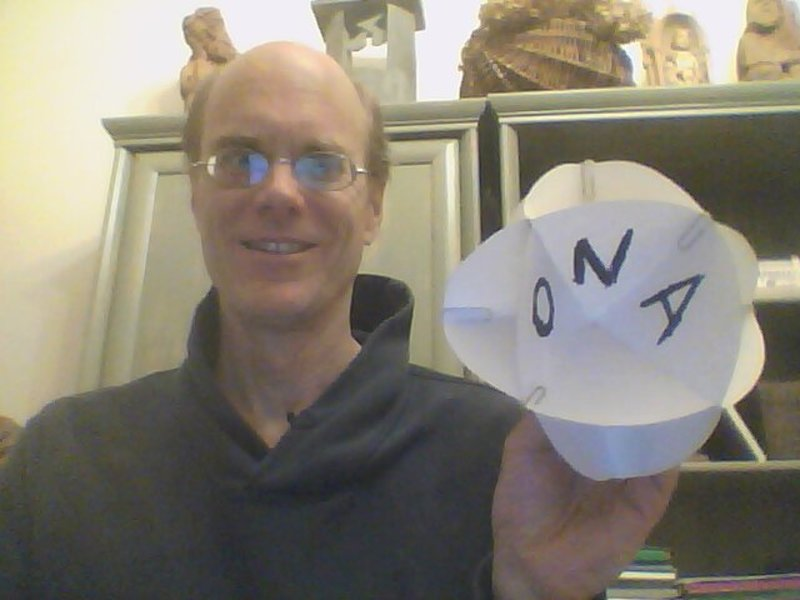# Book: CircleFolding

See: Math

Bradford, thank you for writing about circle folding. I cut out a circle and folded it. I found some of your web pages: http://analyzer.depaul.edu/paperplate/Full%20Circle.htm http://wholemovement.com You are a wonderful artist, creative geometer and a true pioneer. I expect to fold more circles and to relate that to some insights I'm developing.

Dear Bradford and all,

Every so often I would like to do some circle folding and share my findings. Thank you, Bradford, for opening up this very new way of thinking for me.

As I wrote, I sent my niece Ona a circle folding link for her birthday: http://wholemovement.com/how-to-fold-circles I made a "sphere" with her name on it and I sent her this photo:As I mentioned, the surprise for me was that the sphere was so taut after I assembled the four circles with paper clips. Another surprise was that I would need to use paper clips. And then I was curious what I had actually built. I counted 14 holes. I realized that 8 of the holes had 3 sides and 6 of the holes had 4 sides. And they were laid out like the 6 faces and 8 corners of a cube. (Equivalently, the 8 faces and 6 corners of an octahedron). So my "sphere" is basically a cuboctahedron.

Each circle (actually, it's a disk) contributed 2 corners (tetrahedrons). And then, apparently, it also contributed 1 1/2 corners of the "octahedron". They aren't cubical corners in the sense that if I try to mount them on a book, I see that the angles are too acute, they aren't "right angles". (I wonder if Kirby's Martians are left-handed and they call the tetrahedron angle the "left angle".) The sphere can be thought of as two half-spheres and the plane in between is divided into 6 angles, which I see need to be equal, so these "left angles" are 360/6 = 60 degrees. They need to be equal because the side of each "square hole" matches the side of a "triangular hole" and the triangle at the top of the sphere is clearly equilateral.

Putting together the 4 circles creates the 6 "square holes". Basically, each circle contributes 3 "quarter-squares". So 6 x 3/4 = 4. So we see that the circles pull together two different worlds, the "intra-world" of the holes in the circles and the "inter-world" created by the spaces between the circles. This distinction may be very meaningful in the field of "homology" which is a very abstract branch of mathematics that is basically the study of "holes". And it's a very difficult, abstract subject perhaps because the holes "are not there" from the point of view of set theory, so it's hard for set theory to talk about them.

It's also very helpful to be able to build models of the three-dimensional polytopes because I've been thinking a lot about polytopes as they turn out to be central to the distinctions I am looking for, many of which Kirby discusses.

Theoretically, I can think of two ways in which circle folding makes tangible fundamental ideas in math.

Folding the circle at once creates "implicit opposites" very much like i and j, the square roots of negative one. One point that I would make to Steve which I think isn't apparent in his exposition of Clifford Algebras is that there is, a priori, no distinction to be made between the two square roots of negative one as they are both indistinguishable in every way. The distinction i and -i is artificial and misleading if they make us think that one rotation is more preferable or natural than another. Whereas the distinction between +1 and -1 is completely valid because -1 x -1 = 1 whereas 1 x 1 = 1 and so there is a real distinction to be made. So circle folding gives us practice with the implications of such implicit opposites. It models for us the complex plane. Then things like "complex conjugation" become natural. Or looking at the "upper half plane" of the complexes likewise, I imagine. Also, complex multiplication may make more sense. Perhaps we could try to draw a "unit circle" on the circle to consider how that multiplication works. Or perhaps, better yet, we could consider one side of the circle as the In-Side (centered on 0) and the flip side of the paper circle as the Out-Side (centered on infinity). Now we have a representation of the complex plane where 0 and infinity are naturally identifiable and motion away from the unit circle and towards the unit circle is, I expect, equivalent. The two operations involved in complex multiplication - moving towards the center or the edge - and rotating along the edge - then seem completely natural in a way that is unnatural in the "square" Euclidean plane.

Circle folding also comes up, I think, in terms of a more general "sphere-folding" where the sphere can be of any dimension N.

Bradford, here is a picture that I came across today of the Ford circles: https://en.wikipedia.org/wiki/Hardy%E2%80%93Littlewood_circle_method#/media/File:Circumfer%C3%A8ncies_de_Ford.svg So curious!

Parsiųstas iš http://www.ms.lt/sodas/Book/CircleFolding
Puslapis paskutinį kartą pakeistas 2016 birželio 19 d., 14:24